SSC BOARD PAPERS IMPORTANT TOPICS COVERED FOR BOARD EXAM 2024

### Problem Set 5, Chapter 5 - Quadrilaterals Mathematics Part II Solutions for Class 9 Math

##### Problem Set 5
Question 1.

Choose the correct alternative answer and fill in the blanks.

If all pairs of adjacent sides of a quadrilateral are congruent then it is called ....
A. rectangle

B. parallelogram

C. trapezium

D. rhombus

As per the properties of a rhombus:- A rhombus is a parallelogram in which adjacent sides are equal(congruent).

Question 2.

Choose the correct alternative answer and fill in the blanks.

If the diagonal of a square is 12√2 cm then the perimeter of square is ......
A. 24 cm

B. 24√2 cm

C. 48 cm

D. 48√2 cm

Here d= 12√2 = √2 s where s is side of square

Given diagonal = 20 cm

⇒ s =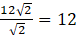Therefore, perimeter of the square is 4s = 4 x 12

= 48cm. (C)

Question 3.

Choose the correct alternative answer and fill in the blanks.

If opposite angles of a rhombus are (2x)° and (3x - 40)° then value of x is .......
A. 100°

B. 80°

C. 160°

D. 40°

As rhombus is a parallelogram with opposite angles equal

⇒ 2x = 3x -40

x= 40°

Question 4.

Adjacent sides of a rectangle are 7 cm and 24 cm. Find the length of its diagonal.

Adjacents sides are 7cm and 24 cm

In a rectangle angle between the adjacent sides is 90°

⇒ the diagonal is hypotenuse of right Δ

By pythagorus theorem

Hypotenuse2 = side2 + side2

Hypotenuse2 = 49 + 576 =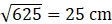length of the diagonal = 25cm

Question 5.

If diagonal of a square is 13 cm then find its side.

given Diagonal of the Square = 13cm

The angle between each side of the square is 90°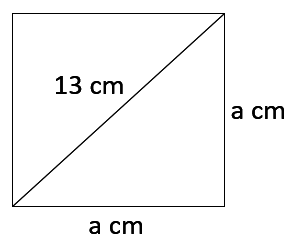Using Pythagoras theorem

Hypotenuse2 = side2 + side2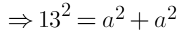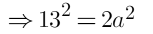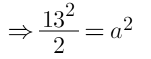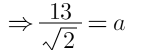Side =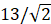cm

Question 6.

Ratio of two adjacent sides of a parallelogram is 3 : 4, and its perimeter is 112 cm. Find the length of its each side.

In a parallelogram opposite sides are equal

Let the sides of parallelogram be x and y

2x+ 2y =112 and given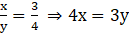⇒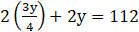⇒7y = 224

y= 32

x= 24

four sides of the parallelogram are 24cm , 32 cm, 24cm, 32cm.

Question 7.

Diagonals PR and QS of a rhombus PQRS are 20 cm and 48 cm respectively. Find the length of side PQ.

According to the properties of Rhombus diagonals of the rhombus bisects each other at 90°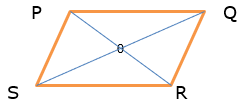In the rhombus PQRS

SO = OQ = 10 cm

PO= OR = 12cm

So in ΔPOQ

∠ POQ = 90°

⇒ PQ is hypotenuse

By Pythagoras theorem,

102 + 242 =PQ2

100 + 576 = PPQ2

676 = PQ2

26cm = PQ Ans

Question 8.

Diagonals of a rectangle PQRS are intersecting in point M. If ∠QMR = 50° then find the measure of ∠MPS.

The figure is given below: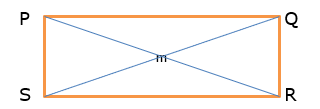Given PQRS is a rectangle

⇒ PS ∥ QR (opposite sides are equal and parallel)

QS and PR are transversal

So ∠ QMR = ∠ MPS (vertically opposite angles)

Given ∠ QMR = 50°

∴ ∠ MPS = 50°

Question 9.

In the adjacent Figure 5.42, if seg AB || seg PQ, seg AB ≅ seg PQ, seg AC || seg PR, seg AC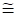seg PR then prove that, seg BC || seg QR and seg BC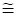seg QR.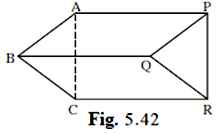Given

AB ∥ PQ

AB ≅ PQ ( or AB = PQ )

⇒ ABPQ is a parallelogram (pair of opposite sides is equal and parallel)

⇒ AP ∥ BQ and AP ≅ BQ……………..1

Similarly given,

AC ∥ PR and AC ≅ PR

⇒ACPR is a parallelogram (pair of opposite sides is equal and parallel)

⇒ AP ∥ CR and AP ≅ CR ……………………2

From 1 and 2 we get

BQ ∥ CR and BQ ≅ CR

Hence BCRQ is a parallelogram with a pair of opposite sides equal and parallel.

Hence proved.

Question 10.

In the Figure 5.43, ABCD is a trapezium. AB || DC. Points P and Q are midpoints of seg AD and seg BC respectively.

Then prove that, PQ || AB and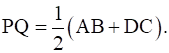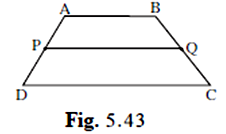Given AB ∥ DC

P and Q are mid points of AD and BC respectively.

Construction :- Join AC

The figure is given below: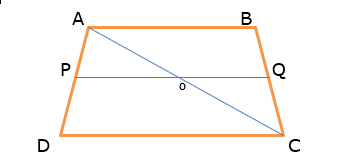P is mid point of AD and PQ is ∥ DC the part of PQ which is PO is also ∥ DC

By mid=point theorem

A line from the mid-point of a side of Δ parallel to third side, meets the other side in the mid-point

⇒ O is mid-point of AC

⇒ PO = 1/2 DC…………..1

Similarly in Δ ACB

Q id mid-point of BC and O is mid –point of AC

⇒ OQ∥ AB and OQ = 1/2 AB………………2

PO + OQ = 1/2 (DC+ AB)

PQ = 1/2 (AB +DC)

And PQ ∥ AB

Hence proved.

Question 11.

In the adjacent figure 5.44, 􀀍ABCD is a trapezium. AB || DC. Points M and N are midpoints of diagonal AC and DB respectively then prove that MN || AB.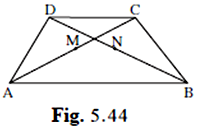Given AB ∥ DC

M is mid-point of AC and N is mid-point of DB

Given ABCD is a trapezium with AB ∥ DC

P and Q are the mid-points of the diagonals AC and BD respectively

The figure is given below: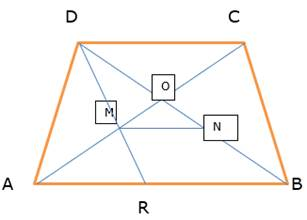To Prove:- MN ∥ AB or DC and

In ΔAB

AB || CD and AC cuts them at A and C, then

∠1 = ∠2 (alternate angles)

Again, from ΔAMR and ΔDMC,

∠1 = ∠2 (alternate angles)

AM = CM (since M is the mid=point of AC)

∠3 = ∠4 (vertically opposite angles)

From ASA congruent rule,

ΔAMR ≅ ΔDMC

Then from CPCT,

AR = CD and MR = DM

Again in ΔDRB, M and N are the mid points of the sides DR and DB,

then PQ || RB

⇒ PQ || AB

⇒ PQ || AB and CD ( ∵ AB ∥ DC)

Hence proved.